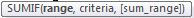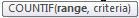### Microsoft Excel - Functions

In this post I would like to talk about two functions COUNTIF and SUMIF. these two functions will be extremely helpful for us to aggregate details very easily. see the examples given below to identify the use.

Sheet value:Fig 1

Find the sum of sales for each sales person. We can use SUMIF function to accomblish this requirement. SUMIF expects three parameter as shown in figure 2. range is the total detailed area where the function has to search for the details, criteria is the value that should find in the given range and the sum range is the range of values to be aggregated for the selected criteria.Fig 2

Formula looks like this =SUMIF(D\$2:E\$10,H\$2,E2:E10) and the result is

 Name Jithesh a b c Sales Amount 95274 476 545 4345

Same way, to find the number of sales done by the person, we can make use of the function countif, an inbuilt function of MS-Excel. It expects two parameter, first is range or the complete details set in the excel sheet and the second is the search criteria for which the numbers has to calculate.Fig 3

Formula looks like this =COUNTIF(\$D\$2:\$E\$10,H2) and the result is as shown below

 Name Jithesh a b c Sales count 4 3 1 1